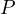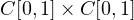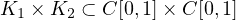Electron. J. Differential Equations, Vol. 2020 (2020), No. 45, pp. 1-15.

Positive solutions for a nonlinear system of fourth-order ordinary differential equations Qiuyue Wang, Lu Yang

Abstract:
In this article, we consider the existence of positive solutions for a nonlinear system of fourth-order ordinary differential equations. By constructing a single conein the product spaceand applying fixed point theorem in cones, we establish the existence of positive solutions for a system in which the nonlinear terms are both superlinear or sublinear. In addition, by the construction of the product conealong with the product formula of fixed point theory on a product cone, we investigate the existence of positive solutions involving nonlinear terms, one uniformly superlinear or sublinear, and the other locally uniformly sublinear or superlinear.

Submitted December 9, 2019. Published May 19, 2020.
Math Subject Classifications: 34B18, 47H11, 47N20.
Key Words: Positive solution; fixed point theory; ordinary differential equation.

Show me the PDF file (322 KB), TEX file for this article.Qiuyue Wang School of Mathematics and Statistics Lanzhou University Lanzhou 730000, China email: wangqy17@lzu.edu.cn Lu Yang School of Mathematics and Statistics Lanzhou University Lanzhou 730000, China email: yanglu@lzu.edu.cn